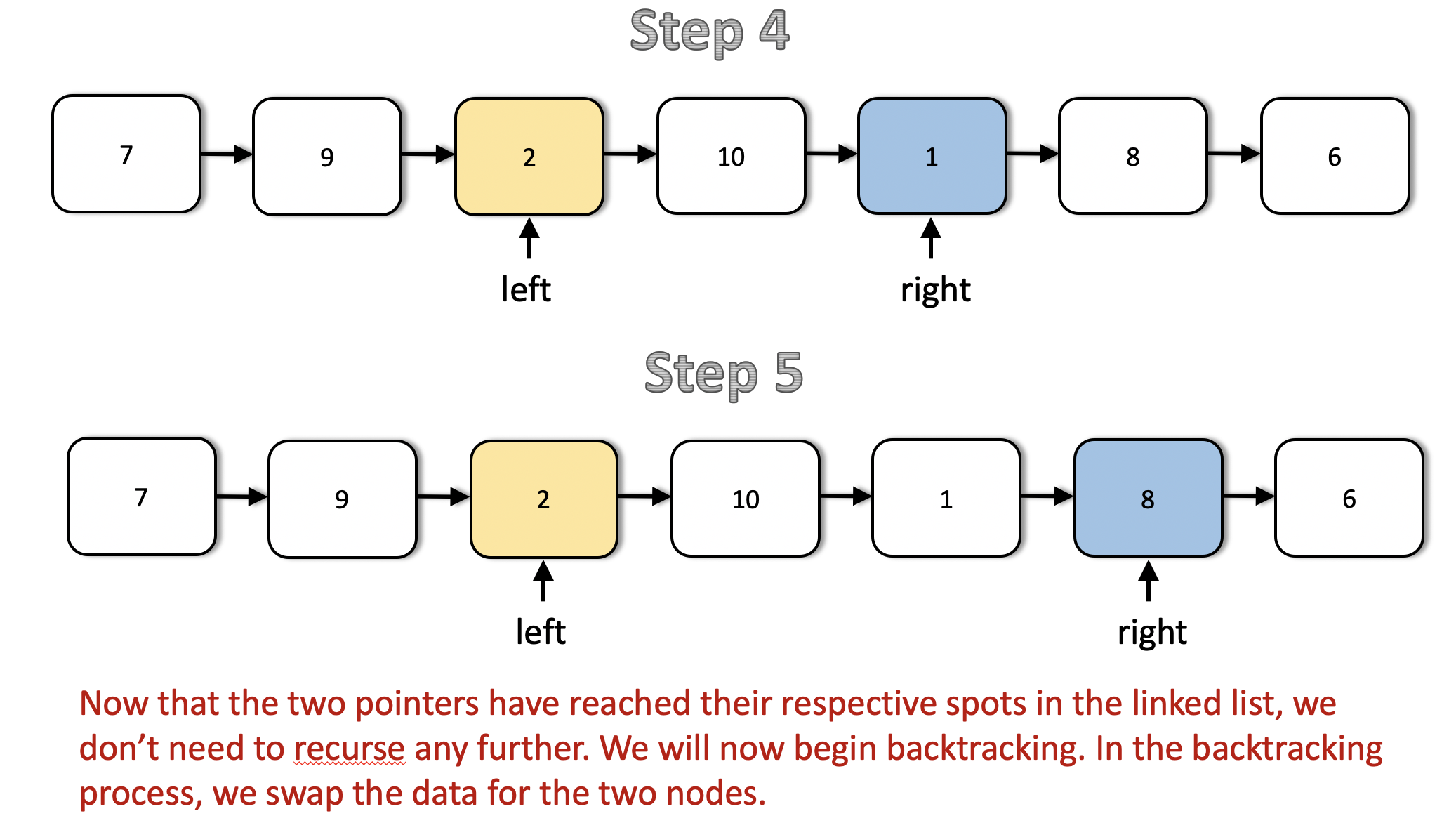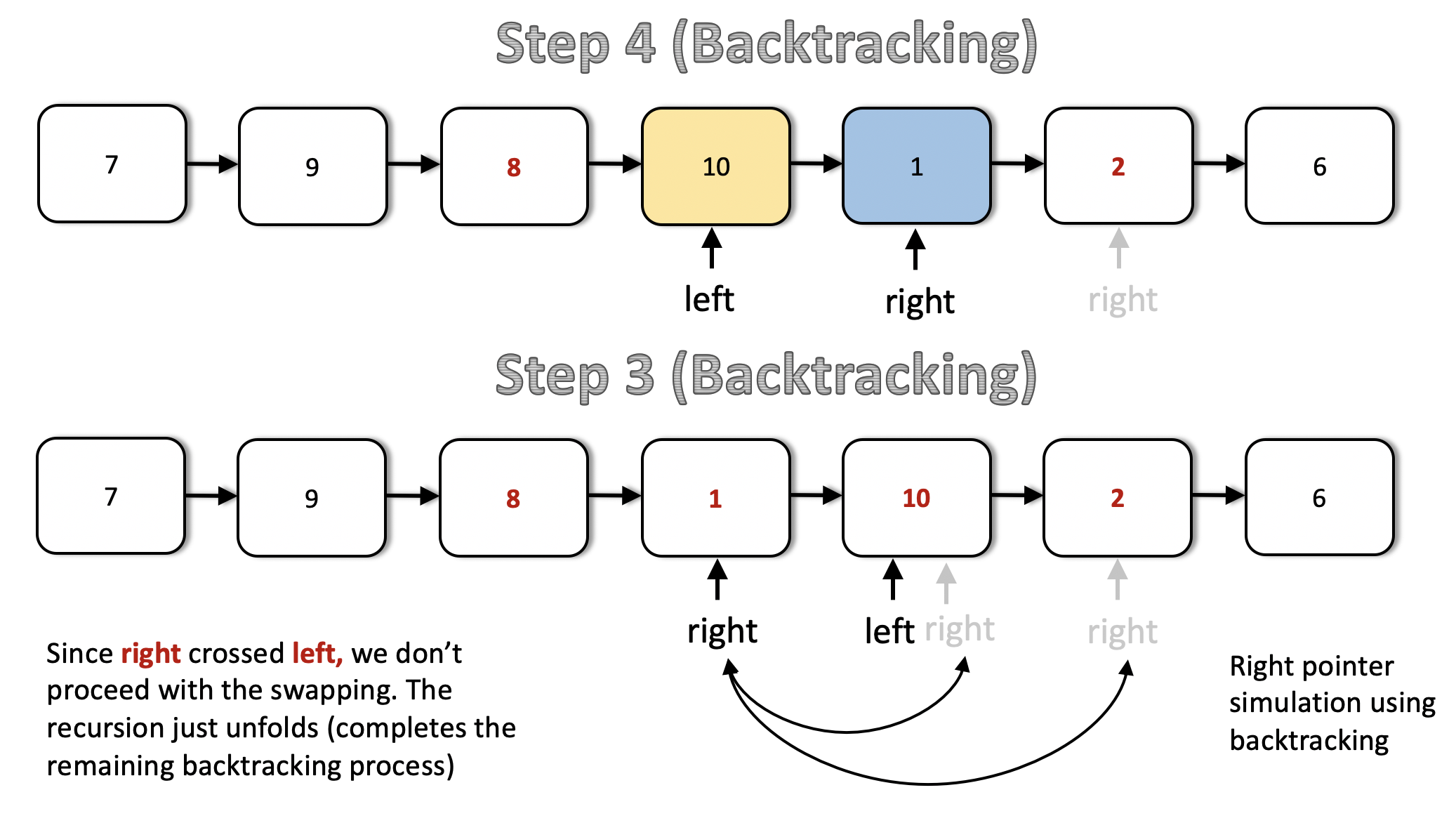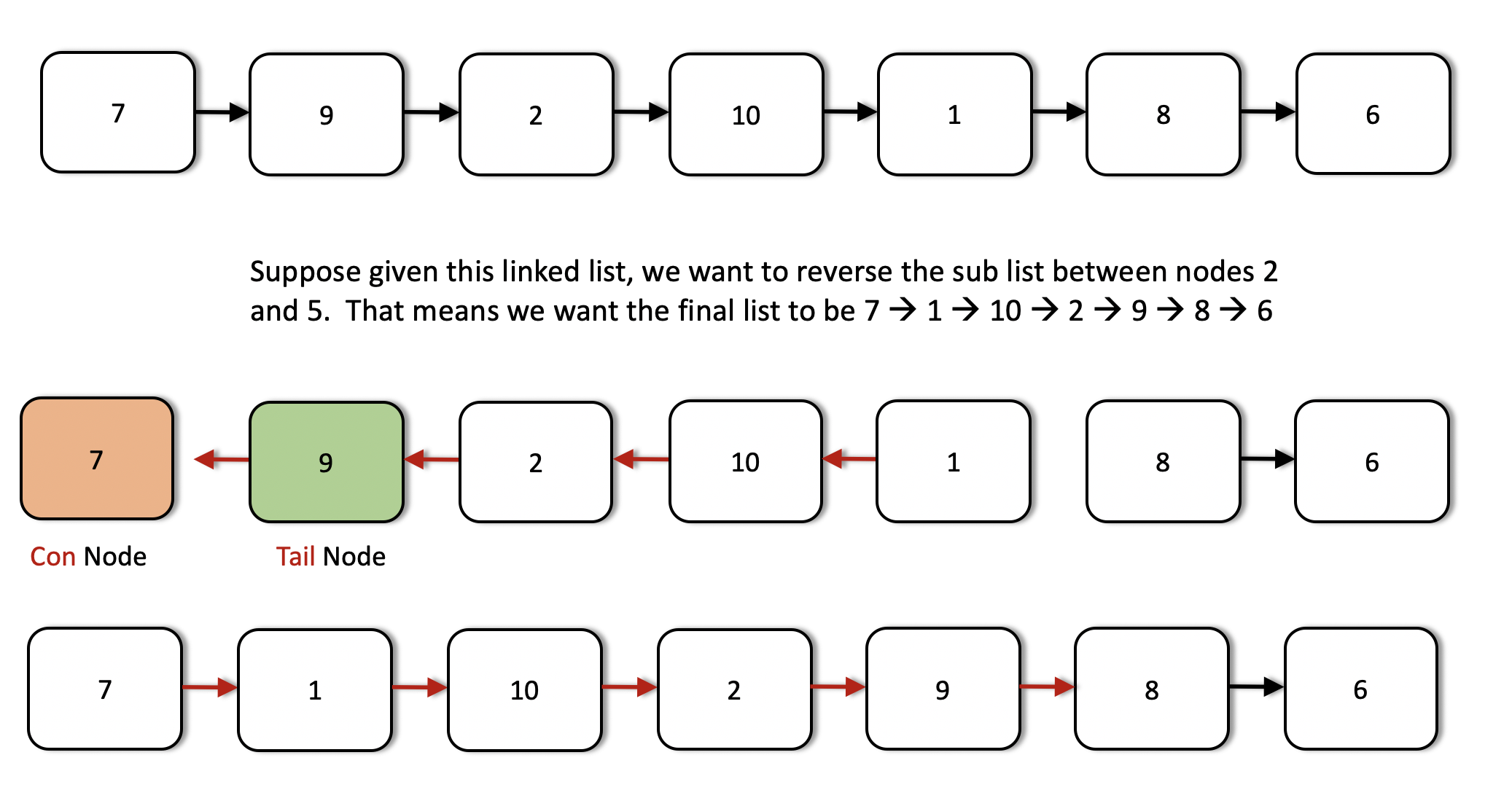## Solution

#### Approach 1: Recursion

Intuition

The idea for linked list reversal using recursion springs from a similar idea that we use for reversing an array. If we want to reverse an array, the huge advantage that we have is the availability of indexes. So, what we can do there is to simply have two pointers, one at the beginning of the array and one at the end. We repeatedly swap elements pointed to by these two pointers and we move both the pointers towards the center of the array. Let's quickly look at this simple algorithm on a sample array before we move on to linked lists.The first approach for reversing a portion of the given linked list is based on the similar idea expressed above. Essentially, we want two different pointers, one at the node from the beginning and another one from the node from the beginning. Once we have such pointers in place, we can repeatedly swap the data between the nodes and progress these pointers towards each other like we saw in the case of an array.

However, we don't have any backward pointers in our linked list and neither do we have any indexes. So, we rely on recursion to simulate the backward pointer. Essentially, the backtracking process in a recursion will help us in simulating the backward movement of the pointer from the node in the linked list towards the center.

Algorithm

1. We define a recursion function that will do the job of reversing a portion of the linked list.
2. Let's call this function recurse. The function takes in 3 parameters: m being the starting point of the reversal, n being the ending point for the reversal, and a pointer right which will start at the node in the linked list and move backwards with the backtracking of the recursion. If this is not clear at the moment, the diagrams that follow will help.
3. Additionally, we have a pointer called left which starts from the node in the linked list and moves forward. In Python, we have to take a global variable for this which get's changed with recursion. In other languages, where changes made in function calls persist, we can consider this pointer as an additional variable for the function recurse.
4. In a recursion call, given m, n, and right, we check if n == 1. If this is the case, we don't need to go any further.
5. Until we reach n = 1, we keep moving the right pointer one step forward and after doing that, we make a recursive call with the value of n decreased by 1. At the same time, we keep on moving the left pointer forward until m == 1. When we refer to a pointer being moved forward, it essentially means pointer.next.
6. So we backtrack as soon as n reaches 1. At that point of time, the right pointer is at the last node of the sublist we want to reverse and the left has already reached the first node of this sublist. So, we swap out the data and move the left pointer one step forward using left = left.next. We need this change to persist across the backtracking process.
7. From there on, every time we backtrack, the right pointer moves one step backwards. This is the simulation we've been mentioning all along. The backward movement is simulated by backtracking.
8. We stop the swaps when either right == left, which happens if the sublist size is odd, or, right.next == left which happens when during the backtracking process for an even sized sublist, the right pointer crosses left. We use a global boolean flag for stopping the swaps once these conditions are met.

Let's look at a series of diagrams explaining the process on a sample linked list. Hopefully, things would be clearer after this.This is the first step in the recursion process. We have a list given to us and the left and the right pointers start off from the head of the linked list. The first step makes a recursive call with updated values of m and n i.e. their values each reduced by 1. Also, the left and the right pointers move one step forward in the linked list.The next two steps show the movement of the left and the right pointers in the list. Notice that after the second step, the left pointer reaches it's designated spot. So, we don't move it any further. Only the right pointer progresses from here on out until it reaches node 6.As we can see, after the step 5, both the pointers are in their designated spots in the list and we can start the backtracking process. We don't recurse further. The operation performed during the backtracking is swapping of data between the left and right nodes.The right pointer crosses the left pointer after step 3 (backtracking) as can be seen above and by that point, we have already reversed the required portion of the linked list. We needed the output list to be [7 → 9 → 8 → 1 → 10 → 2 → 6] and that's what we have. So, we don't perform any more swaps and in the code, we can use a global boolean flag to stop the swapping after a point. We can't really break out of recursion per say.

Complexity Analysis

• Time Complexity: since we process all the nodes at-most twice. Once during the normal recursion process and once during the backtracking process. During the backtracking process we only just swap half of the list if you think about it, but the overall complexity is .
• Space Complexity: in the worst case when we have to reverse the entire list. This is the space occupied by the recursion stack.

Intuition

In the previous approach, we looked at an algorithm for reversing a portion of the given linked list such that the underlying structure doesn't change. We only modified the values of the nodes for achieving the reversal. However, it may so happen that you cannot change the data available in the nodes. In that scenario, we have to modify the links themselves to achieve the reversal.

Essentially, starting from the node at position m and all the way up to n, we reverse the next pointers for all the nodes in between. Let's look at the algorithm for achieving this.

Algorithm

Before looking at the algorithm, it's important to understand how the link reversal will work and what set of pointers will be required for the same. Let's say we have a linked list consisting of three different nodes, A → B → C and we want to reverse the links between the nodes and obtain A ← B ← C.

Suppose we have at our disposal, two pointers. One of them points to the node A and the other one points to the node B. Let's call these pointers prev and cur respectively. We can simply use these two pointers to reverse the link between A and B.

cur.next = prev


The only problem with this is, we don't have a way of progressing further i.e. once we do this, we can't reach the node C. That's why we need a third pointer that will help us continue the link reversal process. So, we do the following instead.

third = cur.next
cur.next = prev
prev = cur
cur = third


We do the above iteratively and we will achieve what the question asks us to do. Let's look at the steps for the algorithm now.

1. We need two pointers, prev and cur as explained above.
2. The prev pointer should be initialized to None initially while cur is initialized to the head of the linked list.
3. We progress the cur pointer one step at a time and the prev pointer follows it.
4. We keep progressing the two pointers in this way until the cur pointer reaches the node from the beginning of the list. This is the point from where we start reversing our linked list.
5. An important thing to note here is the usage of two additional pointers which we will call as tail and con. The tail pointer points to the node from the beginning of the linked list and we call it a tail pointer since this node becomes the tail of the reverse sublist. The con points to the node one before node and this connects to the new head of the reversed sublist. Let's take a look at a figure to understand these two pointers better.6. The tail and the con pointers are set once initially and then used in the end to finish the linked list reversal.

7. Once we reach the node, we iteratively reverse the links as explained before using the two pointers. We keep on doing this until we are done reversing the link (next pointer) for the node. At that point, the prev pointer would point to the node.
8. We use the con pointer to attach to the prev pointer since the node now pointed to by the prev pointer (the node from the beginning) will come in place of the node due after the reversal. Similarly, we will make use of the tail pointer to connect to the node next to the prev node i.e. node from the beginning.

Let's have a look at the algorithm execute on a sample linked list to make the use case for all these pointers clearer. We are given a linked list initially with elements 7 → 9 → 2 → 10 → 1 → 8 → 6 and we need to reverse the list from node 3 through 6.We can see the first few steps of our iterative solution above. The first step shows the initialization of the two pointers and the third step shows us the starting point for the list reversal process.This shows us in detail how the links are reversed and how we move forward after reversing the links between two nodes. This step is done multiple times as shown in the following images.As we can see from the above images, now the two pointers have reached their final positions. We are done reversing the sublist that we were required to do i.e. nodes 3 through 6. However, we still have to fix some connections. The next image explains how we use the tail and con pointers to make the final connections.Complexity Analysis

• Time Complexity: considering the list consists of nodes. We process each of the nodes at most once (we don't process the nodes after the node from the beginning.
• Space Complexity: since we simply adjust some pointers in the original linked list and only use additional memory for achieving the final result.

Analysis written by: @sachinmalhotra1993.